# Addition + multiplication - math problems

#### Number of problems found: 130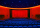Adult tickets in a puppet show sells at P125.05 and children at P99.35. If 25 parents and 70 children watched that show, how much did the show earn?
• Peter 4Peter has salary 45,55 pesos per hour in a regular day and 2,5 times of regular rate over time. He worked 8 hours per regulars day and 4 hours of overtime. Find total amount of pay receive.
• PLUS MINUS product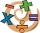A. Deduct 15.728 from the sum of 23.234 and 6.23 B. Add 15.756 to the difference of 26.845 and 4.342 C. Find the product of 0.86 x 0.05 D. Multiply 5.234 by 4.45 E What is the product of 23.97 multiply by 6.36?
• There 19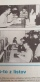There are 19 boys in section A and 23 boys in section B. If 4/7 of them are boy scout, how many boy scouts are there?
• Parentheses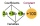Insert parentheses into the expression 2/3 times 5/6 + 3/4 divided by 3/5 so that a. The first operation to be performed is addition and b. The first operation to be performed is division. In both cases evaluate and write results.
• What is 13What is the number sentence? * 1 point 1/2 + 3/4 + 1/4 = A 1/2 - 3/4 - 1/4 = B 1/2 x 3/4 x 1/4 = C 1/2 ÷ 3/4 ÷ 1/4 = D
• 1700 plans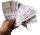1700 plans to budget, 2/5 for rent and 1/6 electricity. What on these two items together?
• A clerkA clerk is paid 45.25 pesos per hour for 40 hours a week. 1.50 times rate for overtime and double the rate for a holiday. How much does the clerk get if he works overtime for 5 hours and 2 hours on holiday?
• Product and sumWhat is the product of two fourths  and the sum of three halves and four?
• The libraryThere are 65 boxes of books. Each box contains 100 books. If each book costs 19.75 how much is the total cost of all the books?
• Nice two numbersWhat two numbers we give a sum of 30 difference of 24 products of 81 and a quotient of 9?
• The space museum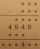The science club is going to the space museum. The club consists of 27 students. If admission costs \$6.50 for children ages 4–11 and \$8.50 for children ages 12–17, how much will it cost for the entire science club's admission to the museum? Science Club S
• Nathan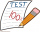Nathan is playing a trivia game. Each correct answer is worth 2 ½ points, and each incorrect answer is worth -¼ points. If he gets 35 questions correct and 32 questions incorrect, how many points does he have? A. 87.5 B. 79.5 C. 75 D. 8
• 30 eggs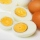There are 30 eggs in a tray. If 1/2 of the tray used 1/5 of it cooked,1/3 kept the refrigerator, how many eggs were left?
• Translate 2Translate the given phrases to mathematical phrases. Thrice the sum of three fifths and two thirds less one half is what number?
• A land 2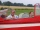A land owner owns two parcels of land measuring 4 2/5 hectares and 6 1/5 hectares. He sells 3/4 of the total land. How many hectares of the land are left?
• Simplify expression with mixedWhen (7¹/3 + 2¾)÷(2 + 2¼ × 1¹/3) is simplified the result is?
• Evaluate 17Evaluate 2x+6y when x=- 4/5 and y=1/3. Write your answer as a fraction or mixed number in simplest form.
• Evaluate fractionsThe difference  of 5 1/2 and 2/3 is added to the product of 5/6 and 1/2
• Sign of a expressionWhat is the sign of expression: minus 18 start fraction 9 divided by 17 end fraction plus left parenthesis minus 18 start fraction 9 divided by 17 end fraction right parenthesis?

Do you have an exciting math question or word problem that you can't solve? Ask a question or post a math problem, and we can try to solve it.

We will send a solution to your e-mail address. Solved examples are also published here. Please enter the e-mail correctly and check whether you don't have a full mailbox.